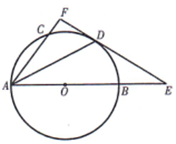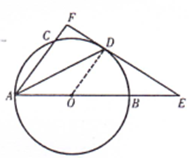$\mathrm{F}$
(1)求证: $A F \perp E F$;
(2) 若 $C F=1, A C=2, A B=4$, 求 $B E$ 的长.【答案】(1) 证明: 连接 0 D ,
\begin{aligned} & \because \mathrm{AD} \text { 平分 } \angle \mathrm{CAB}, \\ & \therefore \angle \mathrm{CAD}=\angle \mathrm{OAD}, \\ & \because \mathrm{OA}=\mathrm{OD}, \\ & \therefore \angle \mathrm{OAD}=\angle \mathrm{ODA}, \\ & \therefore \angle \mathrm{CAD}=\angle \mathrm{ODA}, \\ & \therefore \mathrm{OD} / / \mathrm{AF}, \end{aligned}
$\because \mathrm{EF}$ 为 $\odot 0$ 的切线,
$\therefore \mathrm{OD} \perp \mathrm{EF}$,
$\therefore \mathrm{AF} \perp \mathrm{EF}$.

(2) 解: 由 (1) 得:
$\mathrm{OD} / / \mathrm{AF}$
$\therefore \triangle \mathrm{ODE} \sim \triangle \mathrm{AFE}$
$\therefore \mathrm{OE}: \mathrm{AE}=\mathrm{OD}: \mathrm{AF} \cdot$

\begin{aligned} & \because A \mathrm{AC}=2, \quad \mathrm{CF}=1 \\ & \therefore \mathrm{AF}=3 \\ & \because \mathrm{AB}=4, \\ & \therefore \mathrm{OD}=2, \quad 0 \mathrm{~B}=2 \\ & \therefore 0 \mathrm{E}=0 \mathrm{~B}+\mathrm{BE}=2+\mathrm{x} \\ & \therefore \frac{2+\mathrm{x}}{4+\mathrm{x}}=\frac{2}{3} \quad \ldots \cdots \end{aligned}